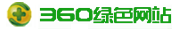1数学模型

1.1管道水击方程

$\text{Error parsing MathML}$

$\text{Error parsing MathML}$

1.2.1水泵的全特性曲线

$WH\left(x\right)=\frac{h}{q{}^{2}+n{}^{2}}$

$WB\left(x\right)=\frac{m}{q{}^{2}+n{}^{2}}$

$\left\{\begin{array}{c}x=\text{a}\text{r}\text{c}\mathrm{tan}\frac{q}{n}\begin{array}{cc}& \end{array}n\ge 0\\ x=\pi +\text{a}\text{r}\text{c}\mathrm{tan}\frac{q}{n}\begin{array}{cc}& \end{array}n<0\end{array}$

$n{}_{s}=\frac{3.65N\sqrt{Q}}{H{}^{3/4}}$

1.2.2力矩平衡方程

$n=n{}_{0}+\frac{m{}_{g}+m{}_{g0}-\left(m+m{}_{0}\right)}{2T{}_{a}}\text{Δ}t$

$n=n{}_{0}-\frac{m+m{}_{0}}{2T{}_{a}}\text{Δ}t$

1.2.3水头平衡方程

$H{}_{p}=C{}_{m2}-C{}_{p1}+\left(B{}_{p1}+B{}_{m2}\right)Q{}_{r}q+C{}_{h}|q|q$

2水泵出口控制阀的理想特性

$Z{}_{1}+\frac{P{}_{1}}{\rho g}+\frac{\alpha {}_{1}Q{}^{2}}{2gA{}_{1}{}^{2}}+H{}_{t}=Z{}_{2}+\frac{P{}_{2}}{\rho g}+\frac{\alpha {}_{2}Q{}^{2}}{2gA{}_{2}{}^{2}}+h{}_{f}+\text{Δ}H$

$P{}_{1}$ $P{}_{2}$ 为进、出水池表面大气压力，由于进、出水池表面大气压力差别较小，可认为$P{}_{1}=P{}_{2}$ ；一般而言，断面行近流速水头$\alpha {}_{1}Q{}^{2}/\left(2gA{}_{1}{}^{2}\right)$ $\alpha {}_{4}Q{}^{2}/\left(2gA{}_{2}{}^{2}\right)$ 很小，可忽略不计。则由式（10）可得

$H{}_{t}=\text{Δ}Z+SQ{}^{2}+\text{Δ}H$

$\tau =\sqrt{\zeta {}_{a}/\zeta }$ ，则控制阀的水头损失可改写为：

$\text{Δ}H=\frac{\text{Δ}H{}_{a}}{Q{}_{a}}\frac{Q{}^{2}}{\tau {}^{2}}$

$S=\frac{H{}_{ta}-\text{Δ}Z-\text{Δ}H{}_{a}}{Q{}_{a}{}^{2}}$

$Q=Q{}_{a}y$

$H{}_{t}=\text{Δ}Z+\left(H{}_{ta}-\text{Δ}Z-\text{Δ}H{}_{a}\right)y{}^{2}+\frac{\text{Δ}H{}_{a}}{\tau {}^{2}}y{}^{2}$

$\tau =y\sqrt{\frac{\text{Δ}H{}_{a}}{H{}_{t}-\text{Δ}Z-\left(H{}_{ta}-\text{Δ}Z-\text{Δ}H{}_{a}\right)y{}^{2}}}$

$\tau =y\sqrt{\frac{\text{Δ}H{}_{a}}{H{}_{ta}-\text{Δ}Z-\left(H{}_{ta}-\text{Δ}Z-\text{Δ}H{}_{a}\right)y{}^{2}}}$

3工程实例分析

Tab.1 Characteristic parameters of pipeline

 管道编号 管径/ m 管长/ m 曼宁糙率 水击波速/ (m·s-1) 管道分段数 调整后的水击波速/(m·s-1) 水击波速的相对误差/ % P1 0.8 23.0 0.012 1103 21 1095.2 0.7 P2 0.7 7.0 0.012 1197 6 1166.7 2.5 P3 1.8 366.0 0.014 1258 291 1257.7 0

Tab.2 Characteristic parameters of pump units

 项 目 指 标 数 值 水泵 高效区扬程 /m 139 130 120 高效区流量 /(m3·s-1) 0.88 1.05 1.21 高效区效率 /% 85.20 86.50 86.15 额定转速 /(r·min-1) 993 GD2 /(kg·m2) 238 配套电机 额定功率 / kW 1800 额定电压 / kV 10 同步转速 /(r·min-1) 993 GD2 /(kg·m2) 878

$\tau =y\sqrt{\frac{0.02}{0.31-0.29y{}^{2}}}$

Tab.3 Extreme pressure of control valve outlet and pipeline

 阀门特性编号 控制阀出口 管路沿线 最大压力/ m 最小压力/ m 最大压力/ m 最小压力/ m 特性曲线1 210.31 60.53 210.31 2.58 特性曲线2 185.32 78.84 185.32 10.45 特性曲线3 159.07 78.85 159.07 11.80 特性曲线4 151.71 78.85 151.71 11.81

4结论

（1）控制阀特性是泵站水力过渡过程的重要影响因素，理想的控制阀特性应为下凹形曲线。因此，在泵站工程的控制阀选型中，应尽量选择特性曲线接近理想下凹形曲线的控制阀；

（2）当控制阀特性采用上凸特性时，管路沿线最大水压很大，甚至超过水泵出口额定压力的1.5倍，易造成管道投资显著增加。因此，在进行控制阀选型时，应避免选择上凸形阀门特性；

（3）控制阀采用下凹形特性对于提升管道中的最小压力也是有利的，可有效抑制管路中负压的产生。

Copyright 2006-2019温州哲成自控阀门有限公司 版权所有 转载本站图文敬请注明来源(哲成阀门 www.zczkvalve.com) 违者必究 本站常驻律师：李成新